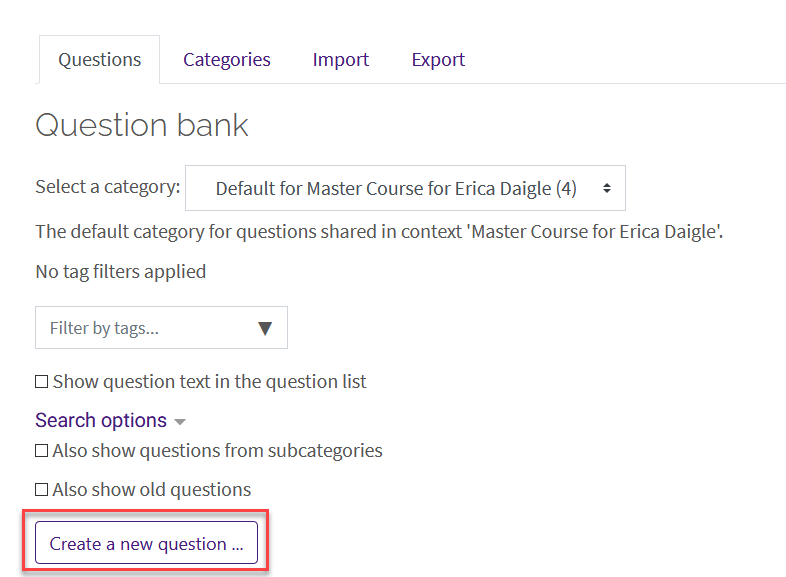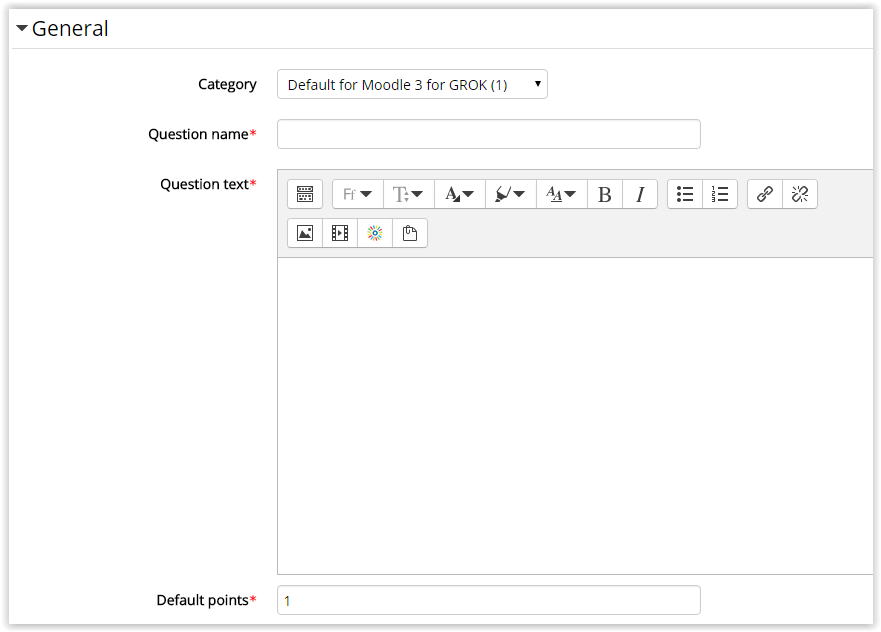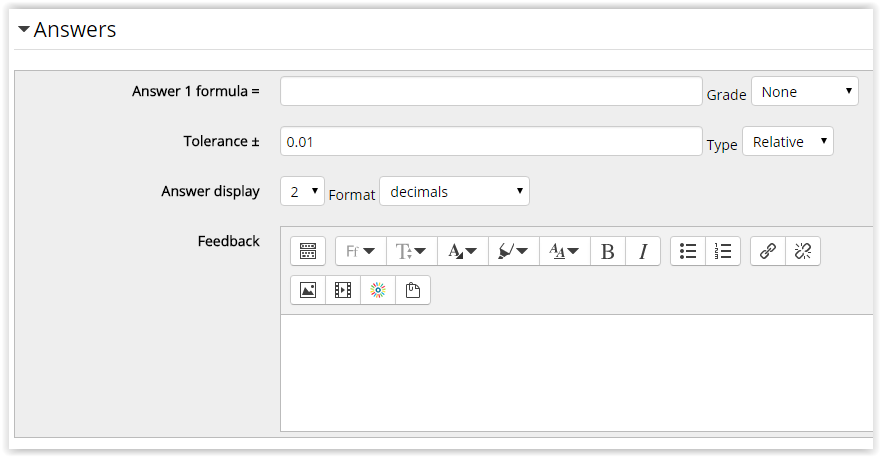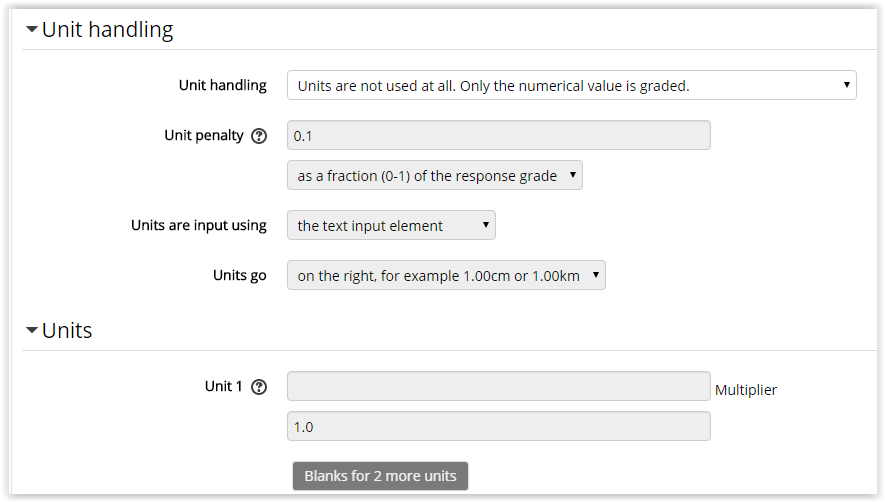### Moodle: Activities & Resources: Quiz Question Types: Calculated Simple

Simple calculated questions offer a way to create individual numerical questions whose response is the result of a numerical formula which contain variable numerical values by the use of wild cards (i.e {x} , {y}) that are substituted with random values when the quiz is taken.

The simple calculated questions offers the most used features of the calculated question with a much simpler creation interface.

#### To Create a Calculated Simple Question

1. Open Moodle and select your course.
2. In the Administration block, click Question Bank then click on Questions.3. Click Create a new question.4. Select Simple Calculated from the sidebar at the left, then click Add at the bottom.
5. Enter the required fields of Question Name, Question Text, and the Points. Optional settings include Category and General feedback.

NOTE: It is suggested that the instructor make sure that the category is correct for organizational reasons and that the question name be something specific (other than Number 1) so that it is easier to find and edit them later.6. The Answer section has optional settings including the Correct answer formula, Grade, Tolerance, Tolerance type, Correct answer shows, Format, and Feedback to be displayed after a student finishes the quiz.7. The Unit section has optional settings that allows the instructor to require if students must have units in their answer.8. Penalty for each incorrect try is required if the quiz is set to adaptive mode (where a student is allowed multiple attempts at a question within a quiz), and will change the percentage deducted for incorrect tries. Hints are optional and can be included after an incorrect try to help the student figure out problems.

Click Save Changes at the bottom when finished.